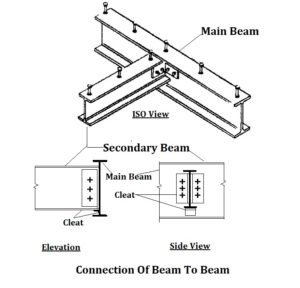# Girder Design Procedure

## Girder Design Procedure:

The design of girder or built up beam is done by trial and error method. The section of the girder is chosen and checked for the stresses. The general steps of girder design are as following.

1. Calculate the bending moment to be opposed by the beam with respect to loads and span of the beam.

Let the maximum bending moment to be opposed is M, and maximum shear force is F.

2. Calculate the suitable measure of modulus of section by the below given formula.

Z = M/P

where P = Permeable bending stress.

3. Select a rolled steel section.

If the modulus of section is small than the required amount of Z, extra cover plates might be included on the both flanges to get required value of Z.The girder sections now checked against bending stresses in extreme fibers.

5. The section of the girder should also be checked for shear force and deflection/

Shear stress of the section is calculated by the below formula

q = F/tw* h

where q = Shear stress

tw =Web thickness,

h = depth of the beam.

The value of q should not cross 94.5 N/mm2. Permeable deflection should not cross L/325 limit.

Where L = Effective span of the beam.